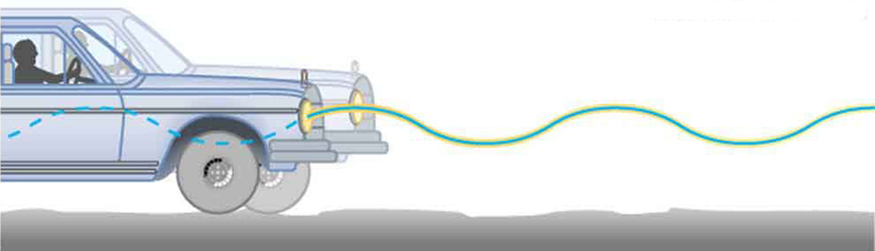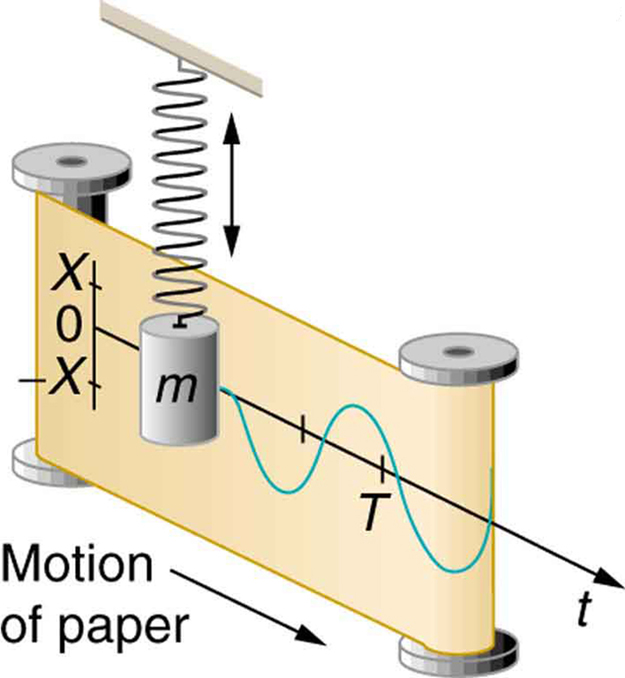# 16.3 Simple harmonic motion: a special periodic motion  (Page 2/7)

 Page 2 / 7

In fact, the mass $m$ and the force constant $k$ are the only factors that affect the period and frequency of simple harmonic motion.

## Period of simple harmonic oscillator

The period of a simple harmonic oscillator is given by

$T=2\pi \sqrt{\frac{m}{k}}$

and, because $f=1/T$ , the frequency of a simple harmonic oscillator is

$f=\frac{1}{2\pi }\sqrt{\frac{k}{m}}.$

Note that neither $T$ nor $f$ has any dependence on amplitude.

## Take-home experiment: mass and ruler oscillations

Find two identical wooden or plastic rulers. Tape one end of each ruler firmly to the edge of a table so that the length of each ruler that protrudes from the table is the same. On the free end of one ruler tape a heavy object such as a few large coins. Pluck the ends of the rulers at the same time and observe which one undergoes more cycles in a time period, and measure the period of oscillation of each of the rulers.

## Calculate the frequency and period of oscillations: bad shock absorbers in a car

If the shock absorbers in a car go bad, then the car will oscillate at the least provocation, such as when going over bumps in the road and after stopping (See [link] ). Calculate the frequency and period of these oscillations for such a car if the car’s mass (including its load) is 900 kg and the force constant ( $k$ ) of the suspension system is $6\text{.}\text{53}×{\text{10}}^{4}\phantom{\rule{0.25em}{0ex}}\text{N/m}$ .

Strategy

The frequency of the car’s oscillations will be that of a simple harmonic oscillator as given in the equation $f=\frac{1}{2\pi }\sqrt{\frac{k}{m}}$ . The mass and the force constant are both given.

Solution

1. Enter the known values of k and m :
$f=\frac{1}{2\pi }\sqrt{\frac{k}{m}}=\frac{1}{2\pi }\sqrt{\frac{6\text{.}\text{53}×{\text{10}}^{4}\phantom{\rule{0.25em}{0ex}}\text{N/m}}{\text{900}\phantom{\rule{0.25em}{0ex}}\text{kg}}}.$
2. Calculate the frequency:
$\frac{1}{2\pi }\sqrt{\text{72.}6/{\text{s}}^{–2}}=1\text{.}{\text{3656}/\text{s}}^{\text{–1}}\approx 1\text{.}{\text{36}/\text{s}}^{\text{–1}}=\text{1.36 Hz}.$
3. You could use $T=2\pi \sqrt{\frac{m}{k}}$ to calculate the period, but it is simpler to use the relationship $T=1/f$ and substitute the value just found for $f$ :
$T=\frac{1}{f}=\frac{1}{1\text{.}\text{356}\phantom{\rule{0.25em}{0ex}}\text{Hz}}=0\text{.}\text{738}\phantom{\rule{0.25em}{0ex}}\text{s}.$

Discussion

The values of $T$ and $f$ both seem about right for a bouncing car. You can observe these oscillations if you push down hard on the end of a car and let go.

If a time-exposure photograph of the bouncing car were taken as it drove by, the headlight would make a wavelike streak, as shown in [link] . Similarly, [link] shows an object bouncing on a spring as it leaves a wavelike "trace of its position on a moving strip of paper. Both waves are sine functions. All simple harmonic motion is intimately related to sine and cosine waves.The bouncing car makes a wavelike motion. If the restoring force in the suspension system can be described only by Hooke’s law, then the wave is a sine function. (The wave is the trace produced by the headlight as the car moves to the right.)The vertical position of an object bouncing on a spring is recorded on a strip of moving paper, leaving a sine wave.

The displacement as a function of time t in any simple harmonic motion—that is, one in which the net restoring force can be described by Hooke’s law, is given by

$x\left(t\right)=X\phantom{\rule{0.25em}{0ex}}\text{cos}\frac{2\mathrm{\pi t}}{T},$

where $X$ is amplitude. At $t=0$ , the initial position is ${x}_{0}=X$ , and the displacement oscillates back and forth with a period $T$ . (When $t=T$ , we get $x=X$ again because $\text{cos}\phantom{\rule{0.25em}{0ex}}2\pi =1$ .). Furthermore, from this expression for $x$ , the velocity $v$ as a function of time is given by:

derivative of first differential equation
why static friction is greater than Kinetic friction
draw magnetic field pattern for two wire carrying current in the same direction
An American traveler in New Zealand carries a transformer to convert New Zealand’s standard 240 V to 120 V so that she can use some small appliances on her trip.
What is the ratio of turns in the primary and secondary coils of her transformer?
nkombo
How electric lines and equipotential surface are mutually perpendicular?
The potential difference between any two points on the surface is zero that implies È.Ŕ=0, Where R is the distance between two different points &E= Electric field intensity. From which we have cos þ =0, where þ is the angle between the directions of field and distance line, as E andR are zero. Thus
sorry..E and R are non zero...
By how much leeway (both percentage and mass) would you have in the selection of the mass of the object in the previous problem if you did not wish the new period to be greater than 2.01 s or less than 1.99 s?
what Is linear momentum
why no diagrams
where
Fayyaz
Myanmar
Pyae
hi
Iroko
hello
Abdu
Describe an experiment to determine short half life
what is science
it's a natural phenomena
Hassan
sap
Emmanuel
please can someone help me with explanations of wave
Benedine
there are seven basic type of wave radio waves, gyamma rays (nuclear energy), microwave,etc you can also search 🔍 on Google :-)
Shravasti
A 20MH coil has a resistance of 50 ohms and us connected in series with a capacitor to a 520MV supply
what is physics
it is the science which we used in our daily life
Sujitha
Physics is the branch of science that deals with the study of matter and the interactions it undergoes with energy
Junior
it is branch of science which deals with study of happening in the human life
AMIT
A 20MH coil has a resistance of 50 ohms and is connected in series with a capacitor to a 250MV supply if the circuit is to resonate at 100KHZ, Determine 1: the capacitance of the capacitor 2: the working voltage of the circuit, given that pie =3.142
Musa
Physics is the branch of science that deals with the study of matter and the interactions it undergoes with energy
Kelly
Heat is transfered by thermal contact but if it is transfered by conduction or radiation, is it possible to reach in thermal equilibrium?
Yes, It is possible by conduction if Surface is Adiabatic
Astronomy
Yeah true ilwith d help of Adiabatic
Kelly
what are the fundamentals qualities
what is physic3
Kalilu
what is physic
Kalilu
Physics? Is a branch of science dealing with matter in relation to energy.
Moses
Physic... Is a purging medicine, which stimulates evacuation of the bowels.
Moses
are you asking for qualities or quantities?
Noman
fundamental quantities are, length , mass, time, current, luminous intensity, amount of substance, thermodynamic temperature.
Shravasti
fundamental quantities are quantities that are independent of others and cannot be define in terms of other quantities there is nothing like Qualities we have only fundamental quantities which includes; length,mass,time, electric current, luminous density, temperature, amount of substance etc
give examples of three dimensional frame of reference
Universe
Noman
Yes the Universe itself
AstronomyByByBy Kimberly NicholsBy Saylor FoundationBy Saylor FoundationBy Marriyam RanaByBy OpenStaxBy Stephen VoronBy Angelica LitoBy Brooke DelaneyBy OpenStax